# Double Integrals over Rectangles

## Calculus 3### 207 Practice Problems

03:59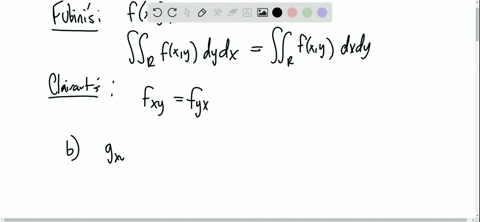Essential Calculus Early Transcendentals

(a) In what way are the theorems of Fubini and Clairaut similar?
(b) If $f(x, y)$ is continuous on $[a, b] \times[c, d]$ and
$$g(x, y)=\int_{a}^{x} \int_{c}^{y} f(s, t) d t d s$$
for $a< x < b, c < y < d,$ show that $g_{x y}=g_{y x}=f(x, y).$

MULTIPLE INTEGRALS
Double Integrals over Rectangles02:01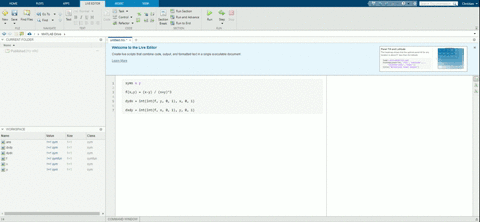Essential Calculus Early Transcendentals

Use your CAS to compute the iterated integrals
$$\int_{0}^{1} \int_{0}^{1} \frac{x-y}{(x+y)^{3}} d y d x \quad \text { and } \quad \int_{0}^{1} \int_{0}^{1} \frac{x-y}{(x+y)^{3}} d x d y$$

MULTIPLE INTEGRALS
Double Integrals over Rectangles02:56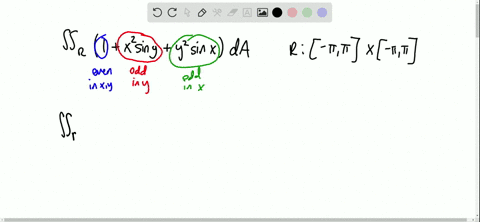Essential Calculus Early Transcendentals

Use symmetry to evaluate the double integral.
$\iint_{R}\left(1+x^{2} \sin y+y^{2} \sin x\right) d A, \quad R=[-\pi, \pi] \times[-\pi, \pi]$

MULTIPLE INTEGRALS
Double Integrals over Rectangles02:25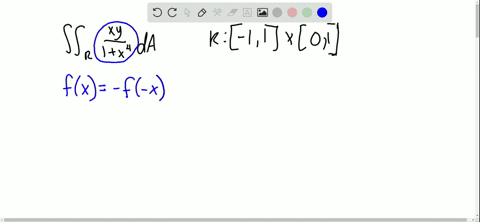Essential Calculus Early Transcendentals

Use symmetry to evaluate the double integral.
$\iint_{R} \frac{x y}{1+x^{4}} d A, \quad R=\{(x, y) |-1 \leqslant x \leqslant 1,0 \leqslant y \leqslant 1\}$

MULTIPLE INTEGRALS
Double Integrals over Rectangles03:01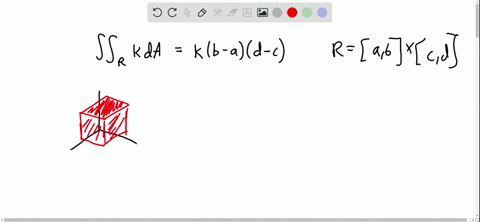Essential Calculus Early Transcendentals

If $f$ is a constant function, $f(x, y)=k,$ and $R=[a, b] \times[c, d],$ show that
$$\iint_{R} k d A=k(b-a)(d-c)$$

MULTIPLE INTEGRALS
Double Integrals over Rectangles04:10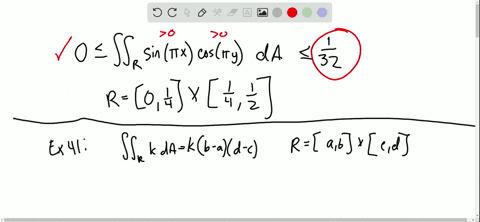Essential Calculus Early Transcendentals

Use the result of Exercise 41 to show that
$$0 \leqslant \iint_{R} \sin \pi x \cos \pi y d A \leqslant \frac{1}{32}$$
where $R=\left[0, \frac{1}{4}\right] \times\left[\frac{1}{4}, \frac{1}{2}\right]$

MULTIPLE INTEGRALS
Double Integrals over Rectangles05:08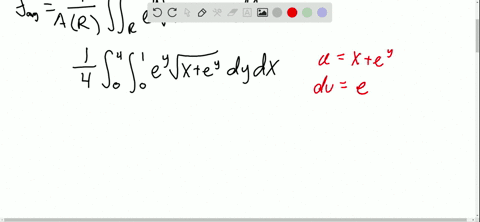Essential Calculus Early Transcendentals

The average value of a function $f(x, y)$ over a rectangle $R$ is defined to be
$$f_{\mathrm{ave}}=\frac{1}{A(R)} \iint_{R} f(x, y) d A$$
(Compare with the definition for functions of one variable in Section $5.4 .$) . Find the average value of $f$ over the given rectangle.
$f(x, y)=e^{y} \sqrt{x+e^{y}}, \quad R=[0,4] \times[0,1]$

MULTIPLE INTEGRALS
Double Integrals over Rectangles02:23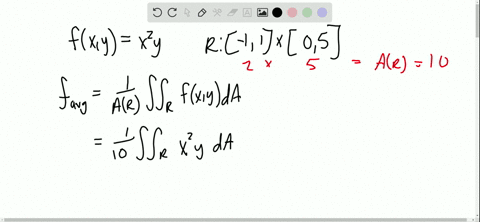Essential Calculus Early Transcendentals

The average value of a function $f(x, y)$ over a rectangle $R$ is defined to be
$$f_{\mathrm{ave}}=\frac{1}{A(R)} \iint_{R} f(x, y) d A$$
(Compare with the definition for functions of one variable in Section $5.4 .$) . Find the average value of $f$ over the given rectangle.
$f(x, y)=x^{2} y$ $R$ has vertices $(-1,0),(-1,5),(1,5),(1,0)$

MULTIPLE INTEGRALS
Double Integrals over Rectangles01:09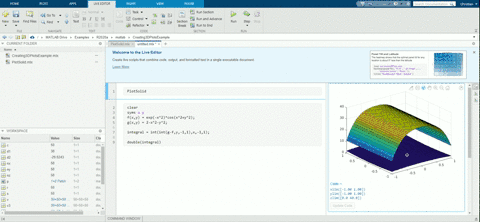Essential Calculus Early Transcendentals

Graph the solid that lies between the surfaces $z=e^{-x^{2}} \cos \left(x^{2}+y^{2}\right)$ and $z=2-x^{2}-y^{2}$ for $|x| \leqslant 1$ $|y| \leqslant 1 .$ Use a computer algebra system to approximate the volume of this solid correct to four decimal places.

MULTIPLE INTEGRALS
Double Integrals over Rectangles01:04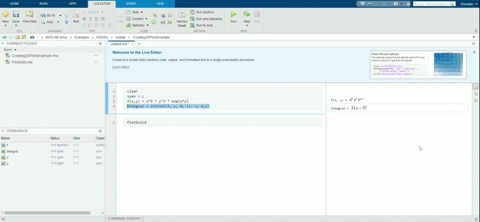Essential Calculus Early Transcendentals

Use a computer algebra system to find the exact value of the integral $\iint_{R} x^{5} y^{3} e^{x y} d A,$ where $R=[0,1] \times[0,1] .$ Then use the CAS to draw the solid whose volume is given by the integral.

MULTIPLE INTEGRALS
Double Integrals over Rectangles03:42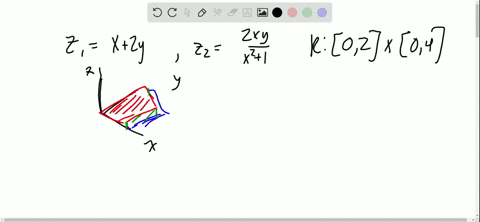Essential Calculus Early Transcendentals

Graph the solid that lies between the surface $z=2 x y /\left(x^{2}+1\right)$ and the plane $z=x+2 y$ and is bounded by the planes $x=0, x=2, y=0,$ and $y=4$ Then find its volume.

MULTIPLE INTEGRALS
Double Integrals over Rectangles04:02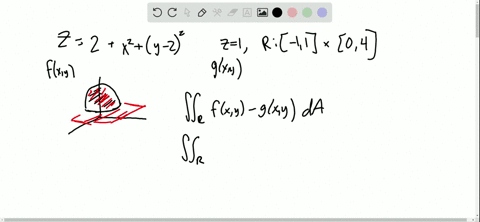Essential Calculus Early Transcendentals

Find the volume of the solid enclosed by the paraboloid $z=2+x^{2}+(y-2)^{2}$ and the planes $z=1, x=1$ $x=-1, y=0,$ and $y=4.$

MULTIPLE INTEGRALS
Double Integrals over Rectangles1 2 3 4 5 ... 18